# Types of Functions | Class 12 Maths

• Difficulty Level : Expert
• Last Updated : 04 Dec, 2020

In this article, we are going to discuss the types of functions. So, Before jumping to the topic firstly you have to know about the functions. So let’s see what are functions.

Note: In the whole article X refers to Domain and Y refers to Codomain.

Hey! Looking for some great resources suitable for young ones? You've come to the right place. Check out our self-paced courses designed for students of grades I-XII

Start with topics like Python, HTML, ML, and learn to make some games and apps all with the help of our expertly designed content! So students worry no more, because GeeksforGeeks School is now here!

## What’s Function?

Let X and Y be two nonempty sets. A function or mapping f from X into Y written as f: X -> Y is a rule by which each element x ∈ X is associated with a unique element y ∈ Y.

### Domain, Codomain, and Range of Function

The elements of X are called the domain of f and the elements of Y are called the domain of f. The images of the element of X is called the range of which is a subset of Y. The below image demonstrates the domain, codomain, and range of the function.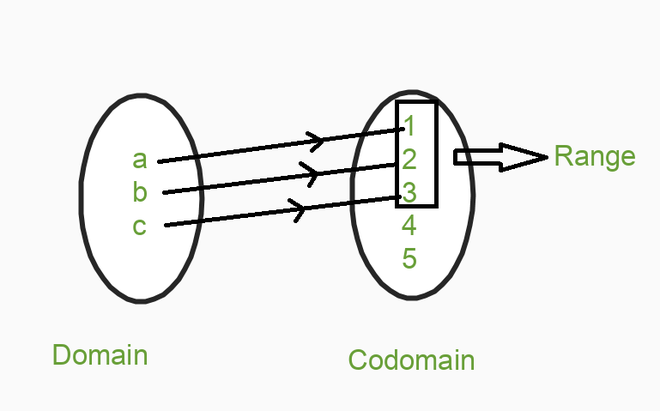The image demonstrates the domain, co-domain, and range of the function. Remember the element which is mapped only they will be counted in the range as shown in the image. If we write the domain, codomain, and range of the above function then

Domain = {a, b, c}

Codomain = {1, 2, 3, 4, 5}

Range = {1, 2, 3}

When will function not exist? The condition when the function will not exist, when all the domain elements are not mapped with the codomain element if any one of the elements will be left to be mapped with codomain then the function will not exist.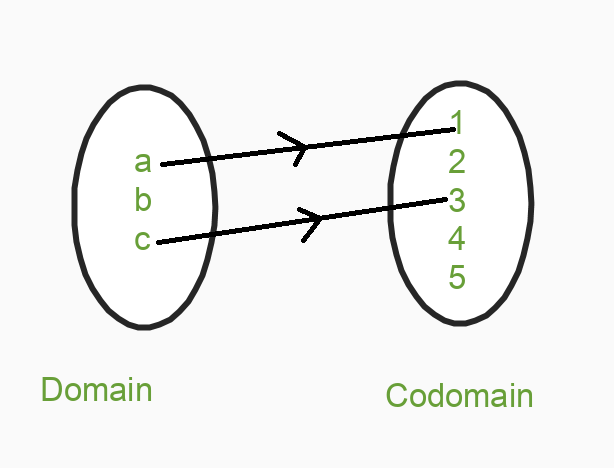From the above example, we can see that all the elements of the domain are not mapped b element is left to be mapped so when this condition arises our function will not exist. Let’s jump to the topic.

## Types of Functions

Basically, there are 6 types of functions.

1. One to One (Injective) function
2. Many to One function
3. Onto (Surjective) Function
4. Into Function
5. One to One Onto Functions (Bijective Function)
6. Many to One Into Function

## One to One (Injective) function

A function f: X -> Y is said to be a one to one function if the images of distinct elements of X under f are distinct. Thus, f is one to one iff f(x1) = f(x2).

Property: A function f: A -> B is one to one if for any f(x1) = f(x2) means  x1 = x2, i.e, image of distinct element of A under f mapping (function) are distinct.

Condition to be One to One function: Every element of the domain has a single image with codomain after mapping.

### Sample Examples on One to One (Injective) function

Example 1: Taking f(x) = 2x + 3, putting 1, 2, 1/2 in place of x.

• So the Domain = {1, 2, 1/2}
• Codomain = (5, 7, 4}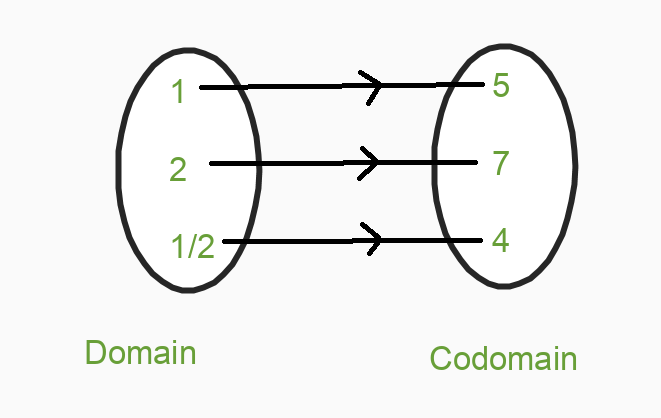From the above image, we can conclude that our function f(x) is One to One because every element of the domain having a single image.

Example 2: Check whether the function is  one to one or not: f(x) = 3x – 2

Solution:

To check the function is one to one or not, we have to check that elements of the domain are having only a single pre-image in codomain or not. For checking, we can write the function as,

f(x1) = f(x2

3x1 – 2 = 3x2 – 2

3x1 = 3x2

x1 = x2

Since both x1 =  x2 means that elements of the domain having a single pre-image in its codomain. Hence the function f(x) = 3x – 2 is one to one function.

Example 3: Check whether the function is  one to one or not: f(x) = x2 + 3.

Solution:

To check whether the function is One to One or not, we will follow the same procedure. Now let’s check, we can write the function as,

f(x1) = f(x2

(x1)2 + 3 = (x2)2 + 3

(x1)2 = (x2)2

Since (x1)2 ≠ (x2)2

Hence the function f(x) = x2 + 3 is not one to one function.

Example 4: If N -> N, f(x) = 2x + 1 then check whether the function is injective or not?

Solution:

In question N -> N, where N belongs to Natural Number, which means that the domain and codomain of the function is a natural number. For checking whether the function is injective or not, we can write the functions as,

Let, f(x1) = f(x2)

2x1 + 1= 2x2 + 1

2x1 = 2x

x1 = x2

Since x1 = x2, means all elements of the domain are mapped with a single element of the codomain. Hence function f(x) = 2x + 1 is Injective (One to One).

Example 5: If f: R -> R by f(x) = 1/x. Then check whether the given function is One to One or not?

Solution:

In question R -> R, where R belongs to Non-Zero Real Number, which means that the domain and codomain of the function are non zero real number. We will check this too as we had done the above question. We can write the function as,

f(x1) = f(x2)

1/x1 = 1/x2

x1 = x2

Since x1 = x2, means all elements of the domain are mapped with a single element of the codomain. Hence the function f(x) = 1/x is One to One.

## Many to One function

If the function is not one to one function then it should be many to one function means every element of the domain has more than one image at codomain after mapping.

Property: One or more elements having the same image in the codomain

Condition to be Many to One function: One or more than one element in the domain having a single image in the codomain.

### Sample Examples on Many to One function

Example 1: f(x) = x2. Check whether the function is Many to One or not?

Solution:

Domain = {1, -1, 2, -2}, let’s put the elements of the domain in the function

f(1) = 12 = 1

f(-1) = (-1)2 = 1

f(2) = (2)2 = 4

f(-2) = (-2)2 = 4

So our Codomain = {1, 4}. After mapping: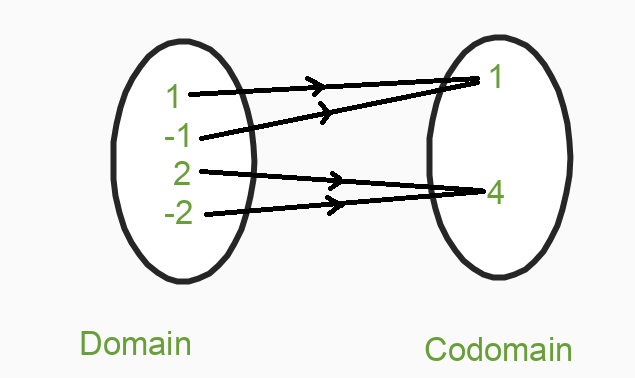From the above mapping, we can see that one than one element of the domain having a single image after mapping. So this is our Many to One function.

Example 2: A = {1, 2, 3, 4}, B = {a, b, c, d, e} function is defined as f = {(1, a), (2, a), (3, b), (4, c)}. Check whether the function is Many to One or not?

Solution:

Let’s represent the function through mapping.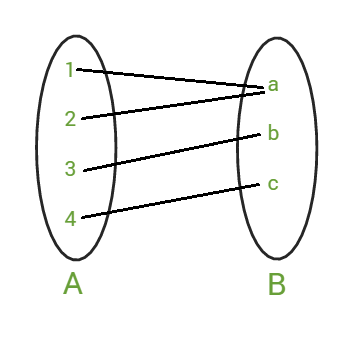As we know the condition of the function to be Many to One is, one or more than one element of domain having same image in the codomain. As we see in the mapping that the element of domain { 1, 2 } is having same image in codomain { a }  after mapping. So the function is Many to One function.

## Onto (Surjective) Function

A function f: X -> Y is said to be an onto function, if every element of Y is an image of some element of set X under f, i.e for every y ∈ Y there exists an element x in X such that f(x) = y.

Properties:

• The range of functions should be equal to the codomain.
• Every element of B is the image of some element of A.

Condition to be Onto function: Range of function should be equal to the codomain.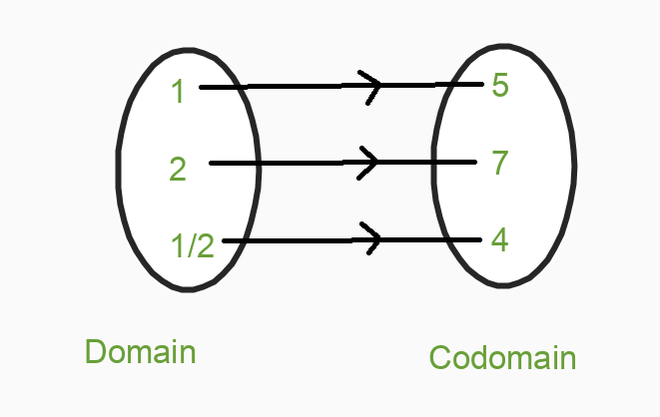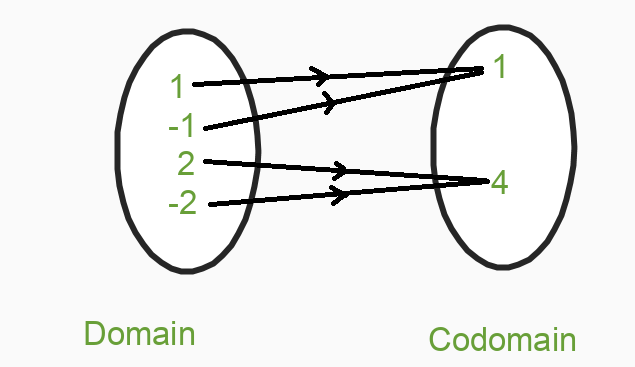As we see in the above two images, the range is equal to codomain means that every element of codomain is mapped with the element of the domain, as we know that elements that are mapped in codomain are known as the range. So these are the examples of the Onto function.

### Sample Examples on Onto (Surjective) Function

Example 1: If R -> R is defined by f(x) = 2x + 1. Then check whether the following function is Onto or not.

Solution:

For checking the function is Onto or not, Let’s first put the function f(x) equal to y

f(x) = y

y = 2x + 1

y – 1 = 2x

x = (y – 1) / 2

Now put the value of x in the function f(x), we get,

f((y – 1) / 2) = 2 . [(y – 1) / 2] +1

Taking LCM 2, we get

= [2(y – 1) + 2] / 2

= (2y – 2 + 2) / 2

= y

Since we get back y after putting the value of x in the function. Hence the given function f(x) = 2x + 1 is Onto function.

Example 2: If f: R – {1} -> R – {1} be defined by f(x) = (x + 1) / (x – 1). Check whether the function is Onto or not.

Solution:

As we did in the first step put function f(x) equal to y and solve

(x + 1) / (x – 1) = y

y (x – 1) = (x + 1)

yx – y = x + 1

Let us transfer x to the left-hand side and y to the right-hand side, we get

yx – x = y + 1

x(y – 1) = y + 1

x = (y + 1) / (y – 1)

Now let’s put the value of x in the function, we get

f((y + 1) / (y – 1)) = [{(y + 1) / (y – 1)} + 1] / [{(y + 1) / (y – 1)} – 1]

= [{(y +1 + y – 1) / (y – 1)} / {(y + 1 – y + 1) / (y – 1)}]

Cancel (y – 1) with (y – 1), we get

= (2y + 1 – 1) /  (2 + y – y)

= 2y / 2

= y

Since we get back y after putting the value of x in the function. Hence the given function f(x) = (x + 1) / (x – 1) is Onto function.

Example 3: If N -> N is defined by f(x) = 3x + 1. Then prove that function f(x) is Surjective?

Solution:

To prove that the function is Surjective or not, firstly we put the function equal to y. Then find out the value of x and then put that value in the function. So let’s start solving it.

Let f(x) = y

3x + 1 = y

3x = y – 1

x = (y – 1) / 3

Now put the value of x in the function f(x), we get

f((y – 1) / 3) = {3 (y – 1) / 3} + 1

= y – 1 + 1

= y

Since we get back y after putting the value of x in the function. Hence the given function f(x) = (3x + 1) is Onto function.

Example 4: If N -> N is defined by f(x) = 1/x. Then check that function f(x) is Onto or not?

Solution:

For every y there exist 1 / y such that,

f(1 / y) = 1 / (1 / y)

then we get only y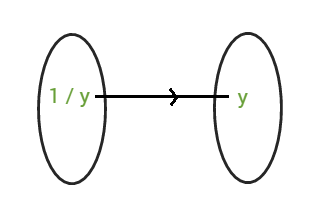means that y belongs to codomain and 1 / y belongs to the domain. So for every 1 / y, we are getting y. Since we get y, this means the function is onto.

Example 5: If N -> N is defined by f(x) = 5x + 3. Then check that function f(x) is Onto or not?

Solution:

To check we have to put the function equal to y

f(x) = y

5x + 3 = y

x = (y – 3) / 5

Put the value of x in the function

f(x) = {5 . (y – 3) / 5} + 3

Cancel 5 with 5, we get

= y – 3 + 3

= y

Since we get back y after putting the value of x in the function. Hence the given function f(x) = 5x + 3 is Onto function.

## Into Function

A function f: X -> Y is said to be an into a function if there exists at least one element or more than one element in Y, which do not have any pre-images in X, which simply means that every element of the codomain are not mapped with elements of the domain.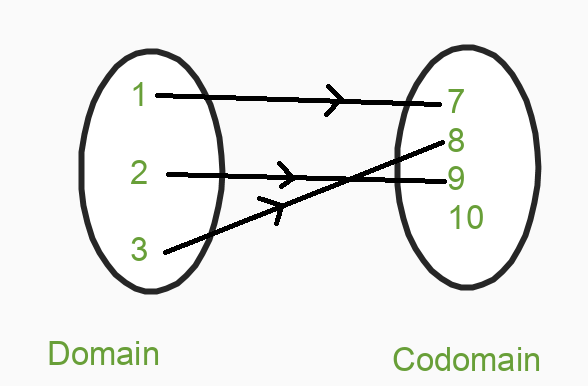From the above image, we can clearly see that every element of codomain is not mapped with elements of the domain means the 10th element of codomain is left unmapped. So this type of function is known as Into functions.

Properties:

• The Range of function is the proper subset of B
• The range of functions should not equal to B, where B is the codomain.

### Sample Examples on Into Function

Example 1: If the sets A = {1, 2, 3}, B = {x, y, z} then the function is defined as f = {( 1, x), (1, y), (2, z)}. Then check whether the function is Into or not?

Solution:

We have f = {(1, x), (1, y), (2, z)}

If we map this function, then we get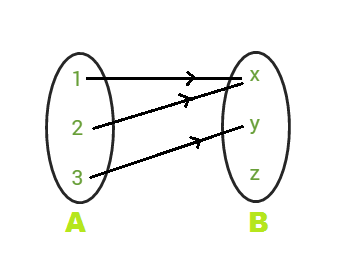In the above mapping we clearly see that the range of function is the proper subset of codomain and also it is not equal to the codomain. Hence we can say that the function is Into function.

Example 2: If the sets A = {1, 2, 3, 4}, B = {a, b, c, d} then the function is defined as f = {(1, d), (2, a), (3, c), (4, b)}. Then check whether the function is Into or not?

Solution:

Let’s first represent the function through mapping.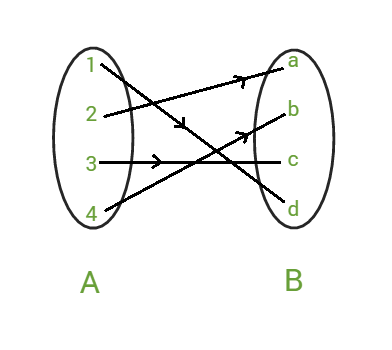As we know the condition for Into function is, that the Range of the function is the proper subset of codomain and also should not equal to the codomain. In the mapping off the function we clearly see that all the elements of the codomain is mapped with elements of domain.

• So, Range of the function = {a, b, c, d}
• Codomain of the function = {a, b, c, d}

Since the Range of the function is equal to codomain of the function. It is proved that the function is not Into function.

## One to One Onto Functions (Bijective Function)

A function f: X-> Y is said to be a bijective function if it is both One to One and Onto.

So let’s see the example of the Bijective function for better understanding.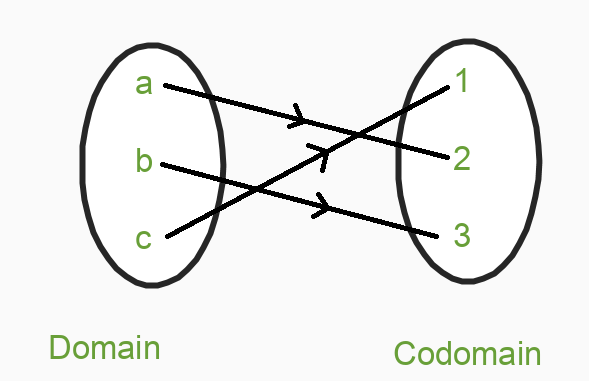Look carefully at the above example, we can see that the function is both One to One and Onto because elements of the domain are having a single image at codomain which is the condition for One to One as we discussed above and other conditions, in which Range is equal to Codomain. So both the conditions for One to One and Onto is satisfied. So our function is One to One Onto.

Properties:

• A function f: A -> B is one to one if for any f(x1) = f(x2) means  x1 = x2, i.e, image of distinct element of A under f mapping (function) are distinct.
• A function f: A -> B is one to one if range of f = B, i.e, f(A) = B
• The range of functions should be equal to the codomain.
• Every element of B is the image of some element of A.

### Sample Examples on One to One Onto Functions (Bijective Function)

Example 1: If A = R – {3} and B = R – {1}. Consider the function f: A -> B defined by f(x) = (x – 2)/(x – 3), for all x ∈ A. Then show that the function f is bijective.

Solution: To show the function is bijective we have to prove the given function both One to One and Onto.

Let’s first check for One to One:

Let x1, x2 ∈  A such that f(x1) = f(x2

Then, (x1 – 2) / (x1 – 3) = (x2 – 2) / (x2 – 3)

(x1 – 2) ( x2 – 3) = (x2 – 2) (x1 – 3)

x1 . x2 – 3x1 – 2x1 + 6 = x1 . x2 – 3x2 -2x1 + 6

-3x1 – 2x2 = -3x2 – 2x1

-3( x1 – x2) + 2( x1 – x2) = 0

-( x1 – x2) = 0

x1 – x2  = 0

⇒ x1 = x2

Thus, f(x1) = f(x2) ⇒ x1 = x2, ∀ x1, x2  ∈ A

So, the function is a One to One

Now let us check for Onto:

Let y ∈ B = R – {1} be any arbitrary element.

Then, f(x) = y

⇒ (x – 2) / (x – 3) = y

⇒ x – 2 = xy – 3y

⇒ x – xy = 2 – 3y

⇒ x(1 – y) = 2 – 3y

⇒ x =  (2 – 3y) / (1 – y) or x = (3y – 2) / (y – 1)

Now put the value of x in the function f(x)

f((3y – 2) / (y – 1)) = { (3y – 2) / (y – 1) } – 2 / { (3y – 2) / (y – 1) – 3 }

= (3y – 2 – 2y + 2) / (3y – 2 – 3y + 3)

= y

Hence f(x) is Onto function. Since we proved both One to One and Onto this implies that the function is bijective.

Example 2: A = {1, 2, 3, 4}, B = {a, b, c, d} then the function is defined as f = {(1, a), (2, b), (3, c), (4, d)}. Check whether the function is One to One Onto or not.

Solution: To check whether the function is One to One Onto or not. We have to check for both one by one. Let’s first represent the function through mapping.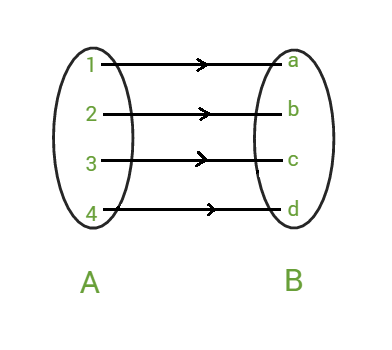Let’s check for One to One:

As we know the condition for One to One that all the elements of the domain are having a single image in the codomain. As we see in the mapping that all the elements of set A are mapped with set B and each having a single image after mapping. So the function is One to One.

Now let’s check for Onto:

As we know the condition for the function to be Onto is that, Range = Codomain means all the elements of codomain are mapped with domain elements, in this case, codomain will equal to the domain. As we see in the mapping that the condition of the function to be Onto is satisfied. So the function is Onto. Since we had proved that the function is both One to One and Onto. Hence function is One to One Onto (Bijective).

## Many to One Into Function

A function f: X-> Y is said to be a Many to One Into function if it is both Many to One and Into.

So let’s see the example of Many to One Into function for better understanding.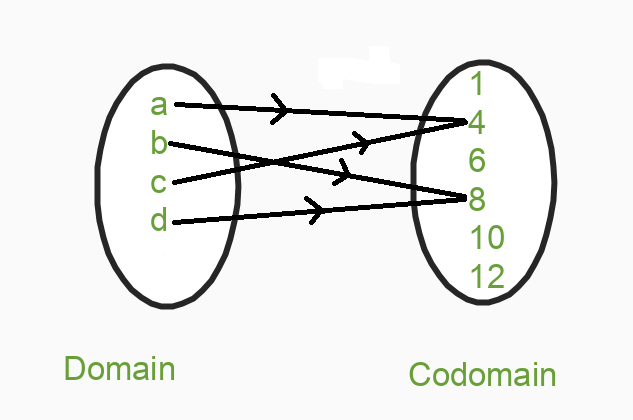So we see in the above example both of the conditions are satisfied. As we see that in codomain {1, 6, 10, 12} these are the elements that are left to be mapped. Firstly the condition that every element of the domain having more than one image at codomain after mapping which is for Many to One as discussed above and other condition, that there exists at least one element or more than one element in Y(Codomain), which do not have any pre-images in X(Domain), which simply means that every element of codomain is not mapped with elements of the domain which is the condition for Into function. So both of the conditions are satisfied that’s why our function is Many to One Into function.

Properties:

• One or more elements having the same image in codomain for Many to One.
• The range of the function is the proper subset of B for into.
• The range of functions should not equal to B for into.

### Sample Examples on Many to One Into Function

Example 1: A = {1, 2, 3, 4}, B = {a, b, c}. Function is defined as f = {(1, a), (2, b), (3, c), (4, c)}.

Solution:

Let’s first represent the function through mapping.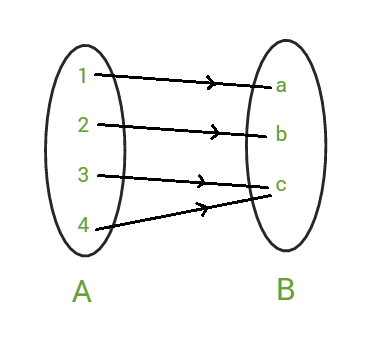To check the function is Many to One Into or not. We have to check for both one by one.

Let’s first check for Many to One function:

As we know the condition for Many to One function is that more than one element of domain should have more same image in codomain. From the above mapping we can see that the elements of A {3, 4 } are having same image in B { c }, so the function is Many to One.

Now let’s check for Into function:

As we know the condition for Into function is that the Range of function should be the subset of codomain and also not equal to codomain. Let’s check both the conditions are satisfied or not.

• Range of function = { a, b, c }
• Codomain of function = { a, b, c }

Range of function = Codomain of function

As we see that the range of function is equal to codomain of the function. Hence we can say that the function is not Into function. As we see that the function is Many to One but not Into, Hence the function is not Many to One Into.

Example 2: A = {1, 2, 3}, B = {a, b, c, d}. Function is defined as f = {(1, a), (2, b), (3, c)}

Solution:

Let’s first represent the function through mapping.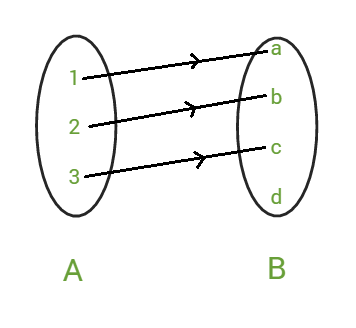As we had done in the above question same we will going to do in this question too.

Let’s first check for Many to One function:

As we know the condition for Many to One function is that more than one element of domain should have more same image in codomain. From the above mapping we can see that every element of A having single image in B , so the function is not Many to One.

Now let’s check for Into function:

As we know the condition for Into function is that the Range of function should be the subset of codomain and also not equal to codomain. Let’s check both the conditions are satisfied or not.

• Range of function = {a, b, c}
• Codomain of function = {a, b, c, d}

Since, Range of function ≠ Codomain of function

As we see that the range of function is not equal to codomain of the function and also Range of the function is the proper subset of codomain. Hence we can say that the function is Into function. As we prove that the function is not Many to One but function is Into,  Since both the conditions are not satisfied. Hence the function is not Many to One Into.

Example 3: A = {1, 2, 3, 4}, B = {a, b, c, d}. Function is defined as f = {(1, a), (2, b), (3, c), (4, c)}.

Solution:

Let’s represent the function through mapping.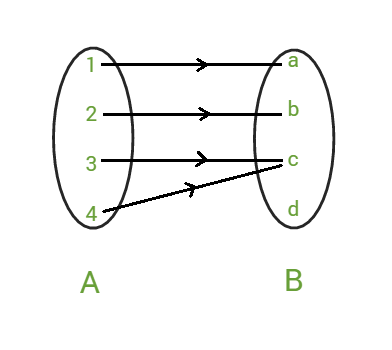To check the function is Many to One Into or not. We have to check for both one by one.

Let’s first check for Many to One function:

As we know the condition for Many to One function is that more than one element of domain should have more same image in codomain. From the above mapping we can see that the elements of A {3, 4 } are having same image in B { c }, so the function is Many to One.

Now let’s check for Into function:

As we know the condition for Into function is that the Range of function should be the subset of codomain and also not equal to codomain. Let’s check both the conditions are satisfied or not.

• Range of function = {a, b, c}
• Codomain of function = {a, b, c, d}

Range of function ≠ Codomain of function

As we check that the range of function is not equal to codomain of the function. Hence we can say that the function is Into function. As we prove that the function is Many to One and Into. Hence the function is Many to One Into.

My Personal Notes arrow_drop_up